Essential Math for Data Science
Do you want more math for data science and machine learning? I just released my book "Essential Math for Data Science"🎉.

Last Update: Sept. 2020

I’m very happy to introduce my work in progress for the book Essential Math for Data Science. It should be available in November 2020.

The goal of the book is to provide an introduction to the mathematics needed for data science and machine learning.

The idea is to use a hands-on approach using examples in Python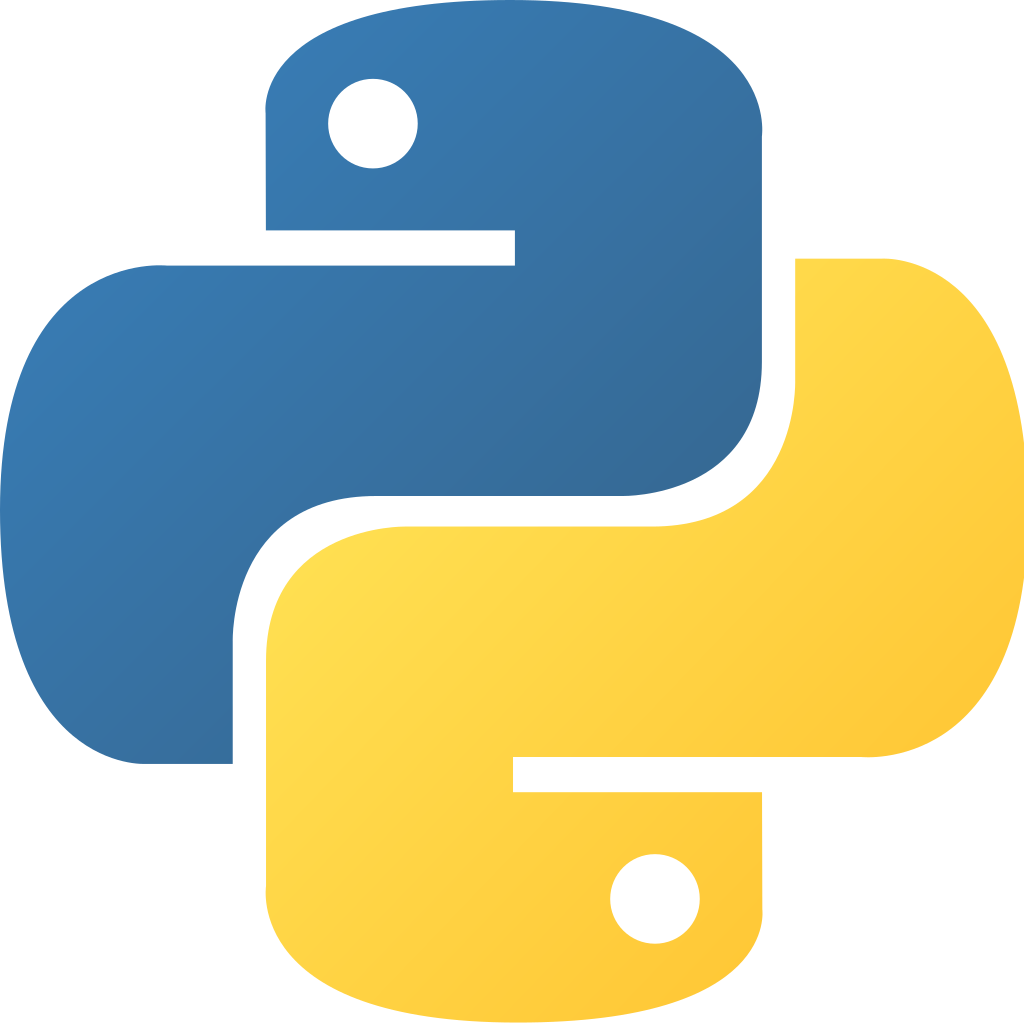, with Numpy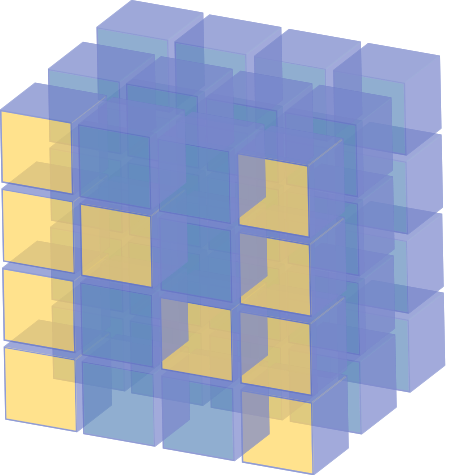, Matplotlib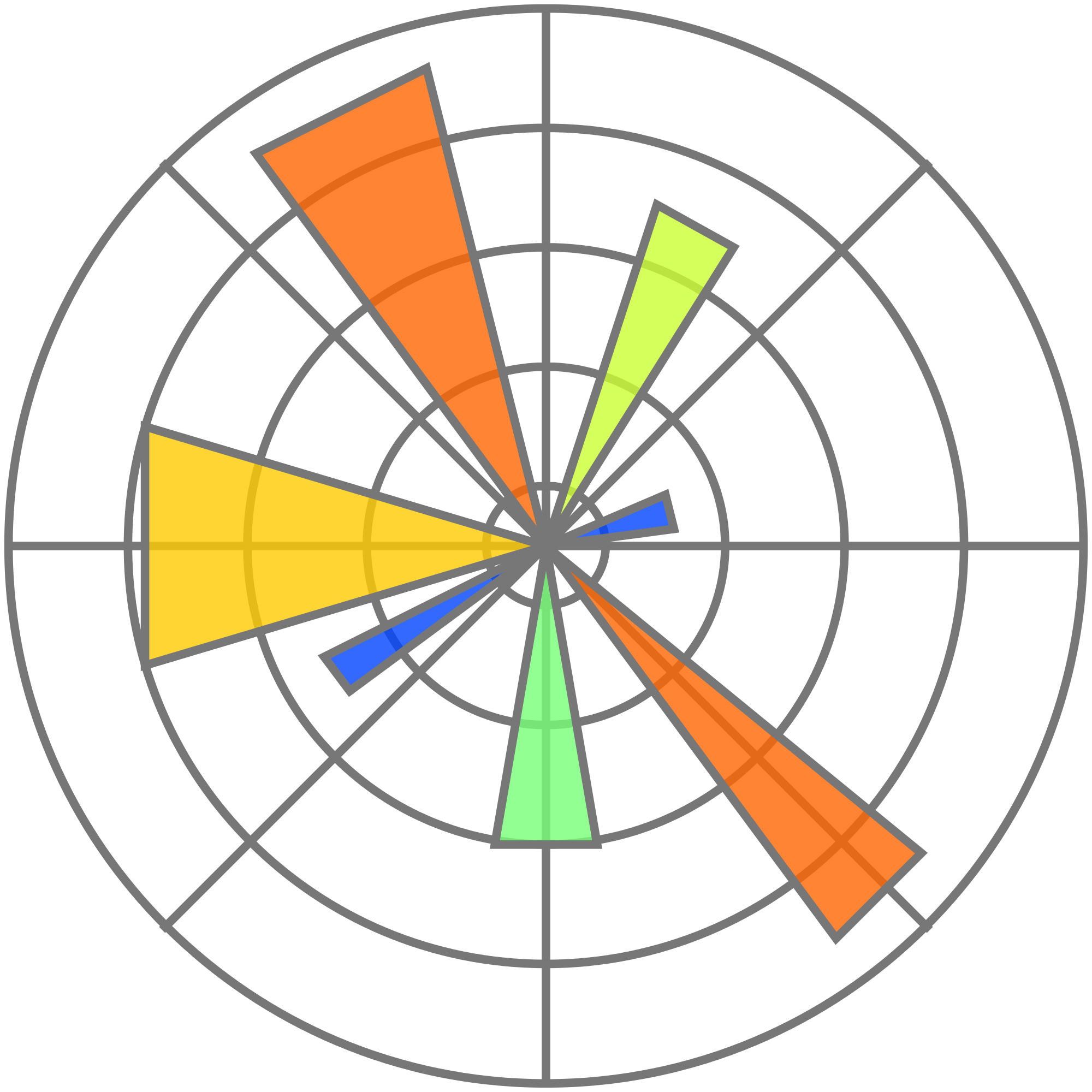, and Sklearn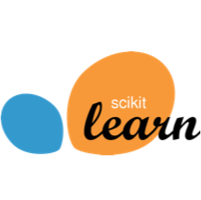to get mathematical insights that will ease the every day life of data engineers or data scientists.

You will be able to experiment on the math concepts and gain intuition through code and visualizations.

What you’ll learn in this book is selected to be actionable knowledge in the context of data science and machine learning / deep learning. The content is more about getting the intuition that will unlock your skills rather than providing mathematical proofs of theorems. It is aimed at people without a deep background in mathematics but who want to understand tools and algorithms used in data science and machine learning, like developers moving towards data science.

Here are some details about the first two parts of the book.

The first three chapters are about the basics, from equations and functions to integrals and derivatives, and they summarize what mathematical bedrock tools you need for data science and the next chapters.

# Part 1. The Basics

## Ch01. Elementary Algebra

Chapter 01 is about equations and functions, with an hands-on project about activation functions in machine learning.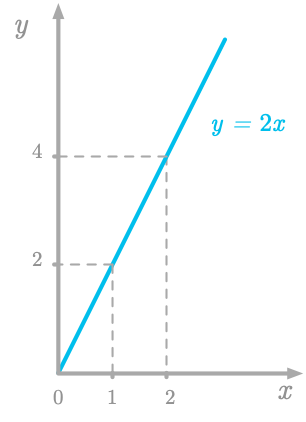Representation of the function $2x$

• Ch01. Elementary Algebra
• 1.1 Variables
• 1.1.1 From Computer Programming to Calculus
• 1.1.2 Unknowns
• 1.1.3 Dependent And Independent Variables
• 1.2 Equations And Inequalities
• 1.2.1 Equations
• 1.2.2 Inequalities
• 1.2.3 Hands-On Project: Standardization and Paris Apartments
• 1.3 Functions
• 1.3.1 From Equations To Functions
• 1.3.2 Computer Programming And Mathematical Functions
• 1.3.3 Nonlinear Functions
• 1.3.4 Inverse Function
• 1.3.5 Hands-On Project: Activation Function

### Ch02. Math On The Cartesian Plane

Chapter 02 is about graphical representation of equations and important concepts like geometric distance (with an hands-on project on the kNN algorithm), or slope and intercept (with an hands-on project on the implementation of the MSE cost function):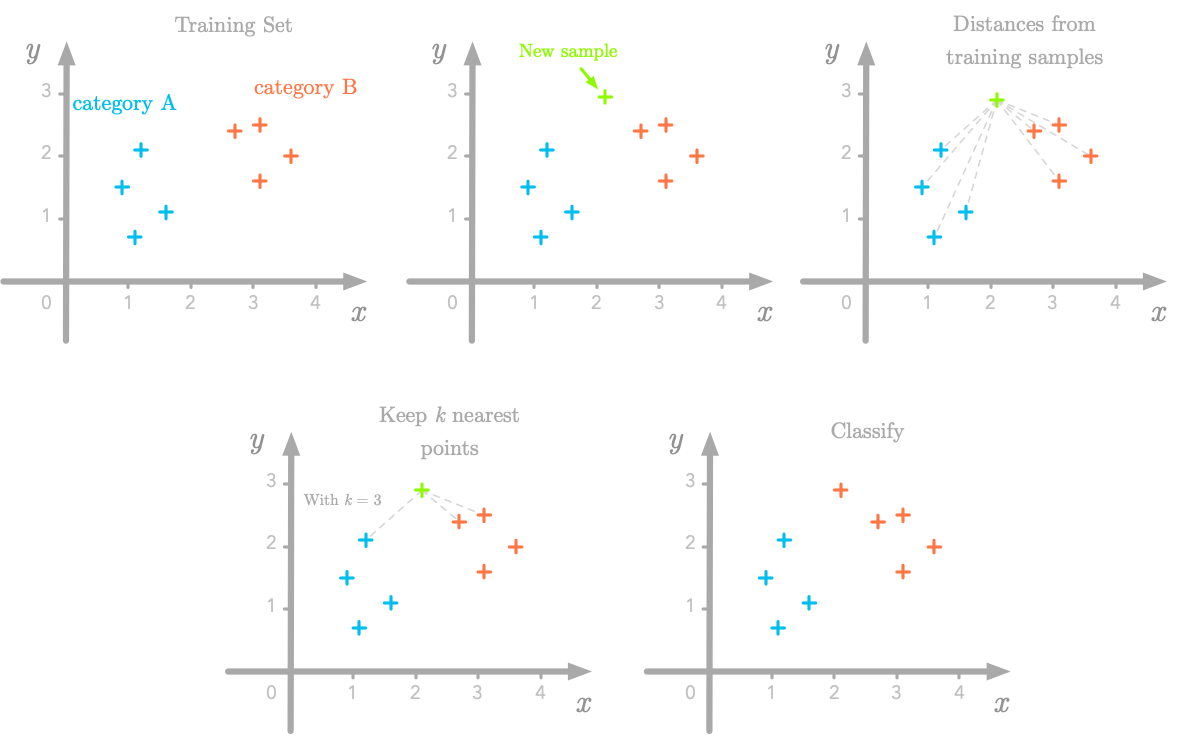Steps of the kNN algorithm

• Ch02. Math On The Cartesian Plane
• 2.1 Coordinates And Vectors
• 2.1.1 Intuition
• 2.1.2 Coordinates
• 2.1.3 Geometric Vectors: Magnitude And Direction
• 2.1.4 Hands-On Project: Images As Model Inputs
• 2.2 Distance formula
• 2.2.1 Definitions
• 2.2.2 Hands-On Project: k-Nearest Neighbors
• 2.3 Graphical Representation of Equations And Inequalities
• 2.3.1 Intuition
• 2.3.2 How To Plot Equations
• 2.3.3 Solving Equations Graphically
• 2.3.4 Inequalities
• 2.4 Slope And Intercept
• 2.4.1 Slope
• 2.4.2 Intercept
• 2.5 Nonlinear functions
• 2.5.1 Definition
• 2.5.2 Function Shape
• 2.6 Hands-On Project: MSE Cost Function With One Parameter
• 2.6.1 Cost function
• 2.6.2 Mathematical Definition of the Cost Function
• 2.6.3 Implementation

### Ch03. Calculus

In Chapter 03, you’ll go through derivatives, partial derivatives and integrals. You’ll see how they relate to data science and machine learning, with the gradient and the area under the ROC curve, for instance.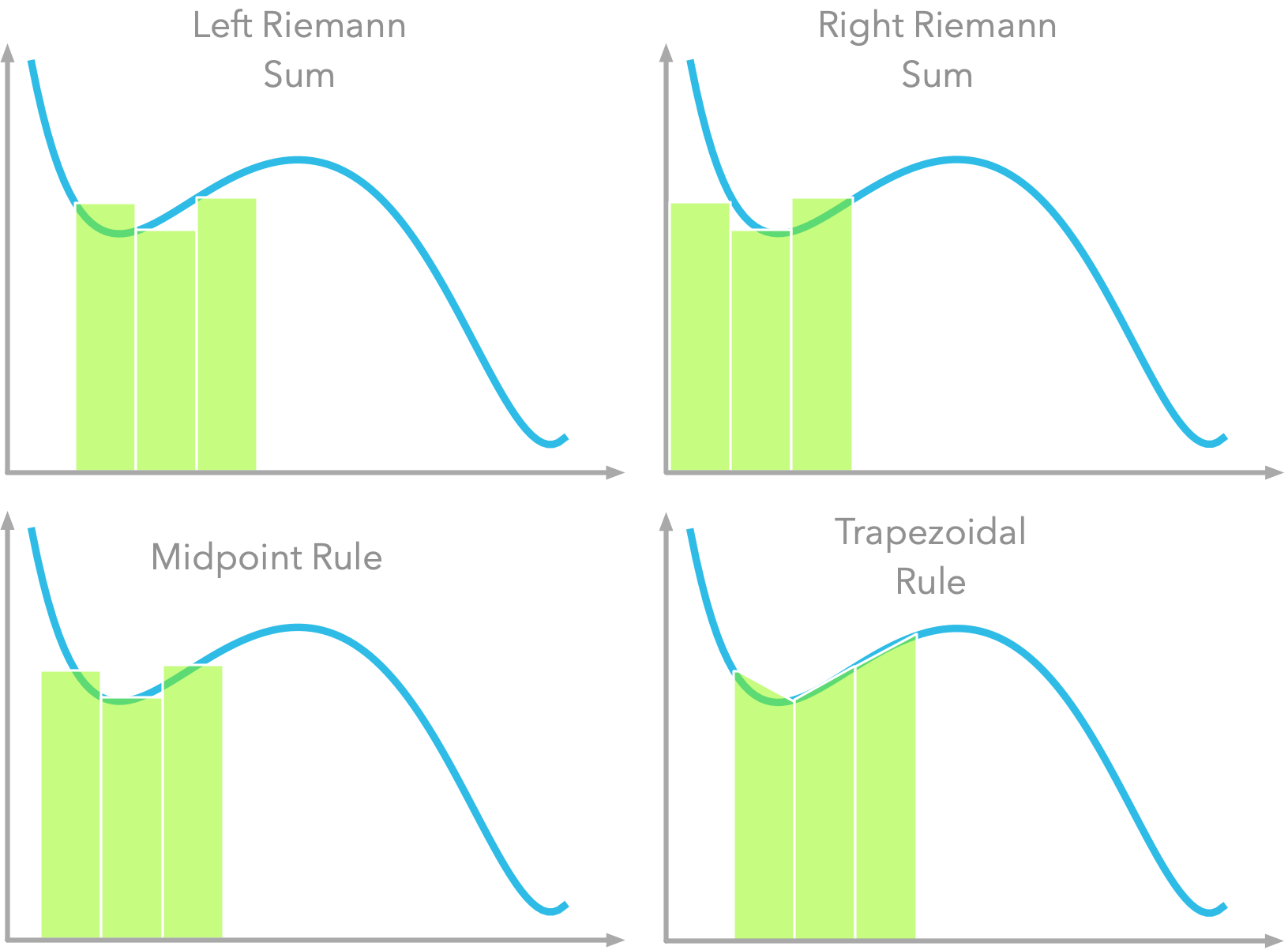Area under the curve

• Ch03. Calculus
• 3.1 Derivatives
• 3.1.1 Insights
• 3.1.2 Mathematical Definition of Derivative
• 3.1.3 Derivatives of Linear And Nonlinear Functions
• 3.1.4 Derivative Rules
• 3.1.5 Hands-On Project: Derivative Of The MSE Cost Function
• 3.2 Integrals And Area Under The Curve
• 3.2.1 Insights
• 3.2.2 Mathematical Definition
• 3.2.3 Hands-On Project: The ROC Curve
• 3.3 Partial Derivatives And Gradients
• 3.3.1 Partial Derivatives
• 3.4 Hands-On Project: MSE Cost Function With Two Parameters
• 3.4.1 The Cost Function
• 3.4.2 Partial Derivatives

## Part 2. Linear Algebra

### Ch04. Scalars and Vectors

Chapter 04 is the first chapter in the central part of the book on linear algebra. It is about scalars and vector. You’ll build the crucial intuition about the relation between geometric vectors and lists of numbers. Then, you’ll start to think in terms of spaces and subspaces. We’ll cover the dot product and the idea of norm, with an example on regularization.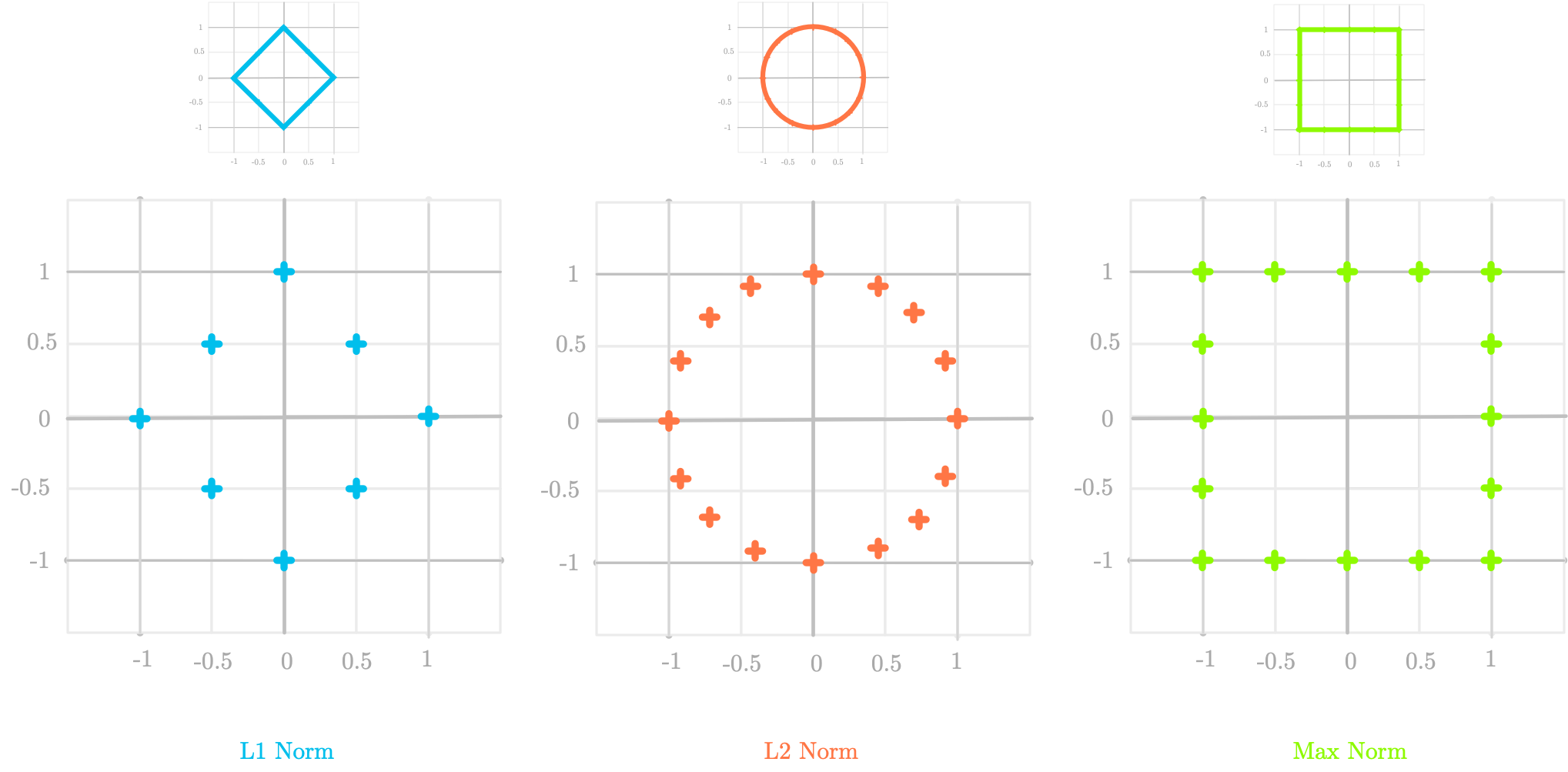Different kind of norms

• Ch04. Scalars and Vectors
• 4.1 Introduction
• 4.1.1 Vector Spaces
• 4.1.2 Coordinate Vectors
• 4.2 Special Vectors
• 4.2.1 Unit Vectors
• 4.2.2 Basis Vectors
• 4.2.3 Zero Vectors
• 4.2.4 Row and Columns Vectors
• 4.2.5 Orthogonal Vectors
• 4.3 Operations and Manipulations on Vectors
• 4.3.1 Scalar Multiplication
• 4.3.3 Using Addition and Scalar Multiplication
• 4.3.4 Transposition
• 4.3.5 Operations on Other Vector Types - Functions
• 4.4 Norms
• 4.4.1 Definitions
• 4.4.2 Examples of Norms
• 4.4.3 Norm Representations
• 4.5 The Dot Product with vectors
• 4.5.1 Definition
• 4.5.2 Geometric interpretation
• 4.5.3 Properties
• 4.5.4 Hands-on Project: Vectorizing the Squared L 2 Norm with the Dot Product
• 4.6 Hands-on Project: Regularization

### Ch05. Matrices and Tensors

In Chapter 05, you’ll learn all you need about matrices. Along with Chapter 04, it makes the foundations of linear algebra, that we’ll use in the next chapters.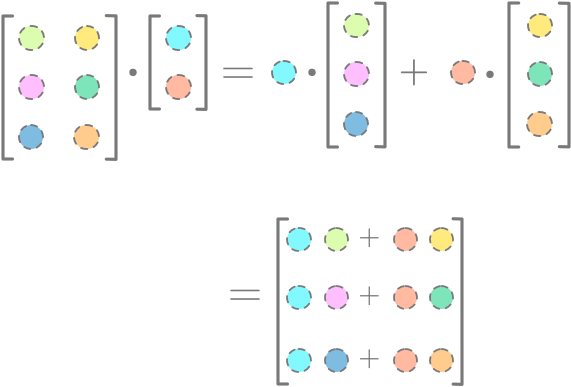Illustration of the dot product between a matrix and a vector

• Ch05. Matrices and Tensors
• 5.1 Introduction
• 5.1.1 From Scalars and Vectors
• 5.1.2 Shapes
• 5.1.3 Indexing
• 5.2 Operations and Manipulations on Matrices
• 5.2.1 Addition and Scalar Multiplication
• 5.2.2 Transposition
• 5.3 The Dot Product with Matrices
• 5.3.1 Vector with Matrices
• 5.3.2 Matrices with Matrices
• 5.3.3 Transpose of a Matrix Product
• 5.4 Special Matrices
• 5.4.1 Square Matrices
• 5.4.2 Diagonal Matrices
• 5.4.3 Identity Matrices
• 5.4.4 Inverse Matrices
• 5.4.5 Orthogonal Matrices
• 5.4.6 Symmetric Matrices
• 5.4.7 Triangular Matrices
• 5.6 Hands-on Projects: Encoding

### Ch06. Span, Linear Dependency, and Space Transformation

In Chapter 04 and 05, we considered vectors and matrices as lists of numbers and geometric representations of these numbers. The goal of Chapter 06 it to go one step ahead and develop the idea of matrices as linear transformations. We’ll also cover the major notions of linear dependency, subspaces and span.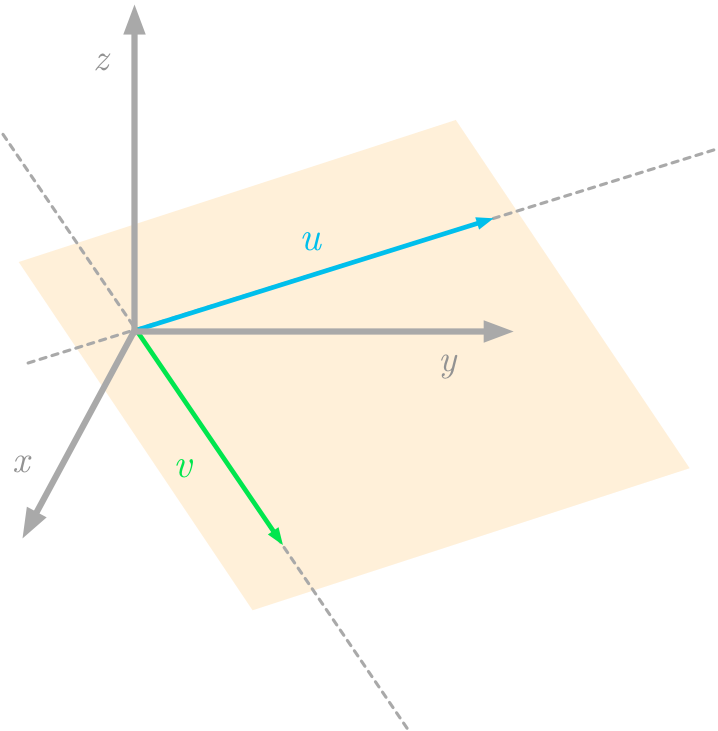Projection

• Ch06. Span, Linear Dependency, and Space Transformation
• 6.1 Linear Transformations
• 6.1.1 Intuition
• 6.1.2 Linear Transformations as Vectors and Matrices
• 6.1.3 Geometric Interpretation
• 6.2 Linear combination
• 6.2.1 Intuition
• 6.2.2 All combinations of vectors
• 6.3 Subspaces
• 6.4 Span
• 6.5 Linear dependency
• 6.5.1 Geometric Interpretation
• 6.5.2 Matrix View
• 6.6 Special Characteristics
• 6.6.1 Rank
• 6.6.2 Trace
• 6.6.3 Determinant
• 6.7 Hands-on Projects
• 6.7.1 Image Filters
• 6.7.2 Matrix Transformation in Linear Regression

### Ch07. Systems of Linear Equations

In this Chapter, we’ll see how you can use matrices and vectors to represent systems of equations and leverage what we learned so far to understand the geometry behind it. We’ll also dive into the concept of projection and see how it relates to systems of equations.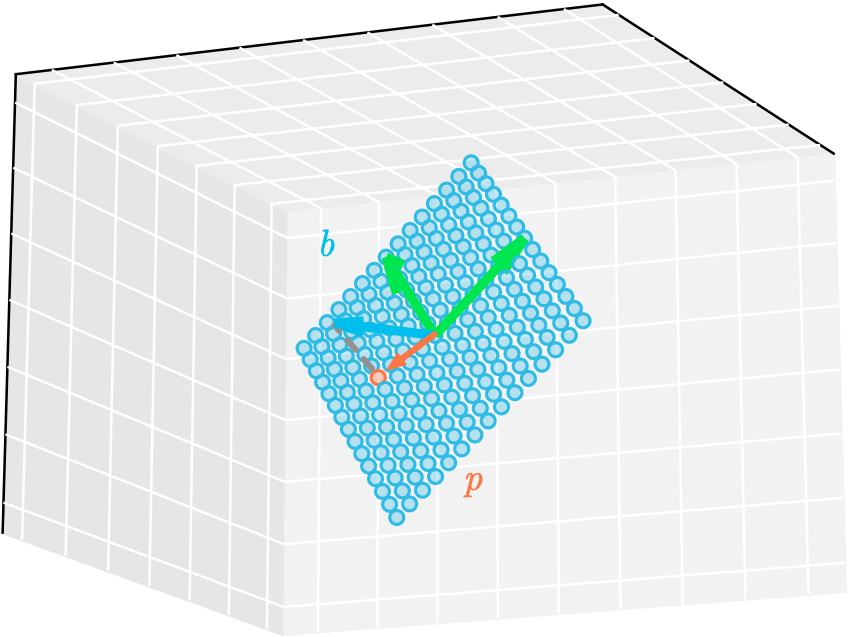Projection

• Ch07. Systems of Linear Equations
• 7.1 System of linear equations
• 7.1.1 Row Picture
• 7.1.2 Column Picture
• 7.1.3 Number of Solutions
• 7.1.4 Representation of Linear Equations With Matrices
• 7.2 System Shape
• 7.2.1 Overdetermined Systems of Equations
• 7.2.2 Underdetermined Systems of Equations
• 7.3 Projections
• 7.3.1 Solving Systems of Equations
• 7.3.2 Projections to Approximate Unsolvable Systems
• 7.3.3 Projections Onto a Line
• 7.3.4 Projections Onto a Plane
• 7.4 Hands-on Project: Linear Regression Using Least Squares Approximation

### Ch08. Eigenvectors and Eigenvalues

In Chapter 08, we’ll use many linear algebra concepts from previous chapters to learn about a major topic: eigendecomposition. We’ll develop intuition about change of basis to understand it, and see its implication in data science and machine learning.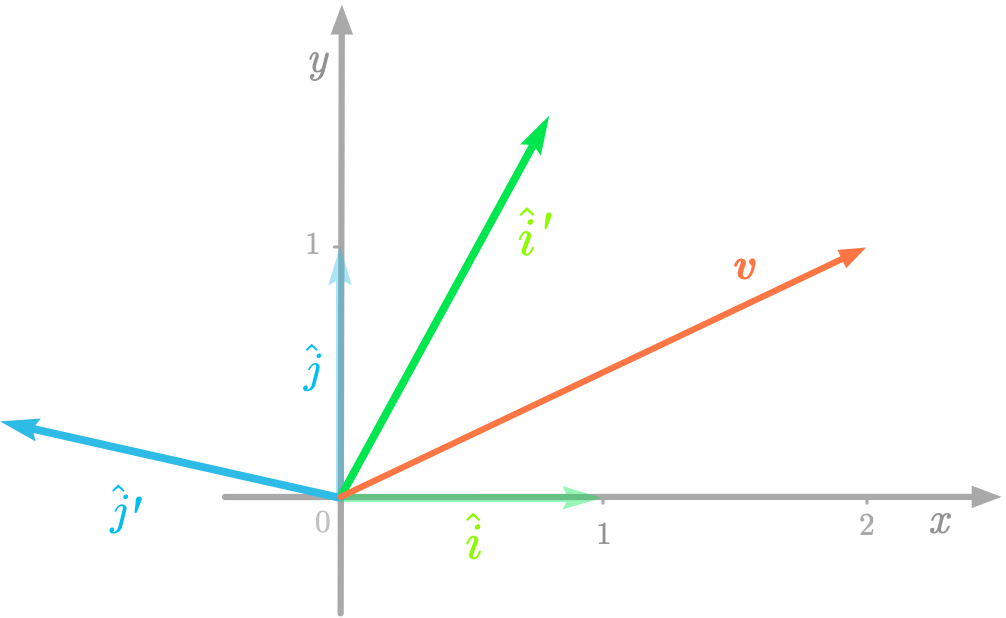Projection

• Ch08. Eigenvectors and Eigenvalues
• 8.1 Eigenvectors and Linear Transformations
• 8.2 Change of Basis
• 8.2.1 Linear Combinations of the Basis Vectors
• 8.2.2 The Change of Basis Matrix
• 8.2.3 Example: Changing the Basis of a Vector
• 8.3 Linear Transformations in Different Bases
• 8.3.1 Transformation Matrix
• 8.3.2 Transformation Matrix in Another Basis
• 8.3.3 Interpretation
• 8.3.4 Example
• 8.4 Eigendecomposition

### Ch09. Singular Value Decomposition

Chapter 09 is the last chapter of Part 2 on linear algebra. You’ll see what is the Singular Value Decomposition or SVD, how it relates to eigendecomposition, and how it can be understood geometrically. We’ll see that it is a great method to approximate a matrix with a sum of low rank matrices.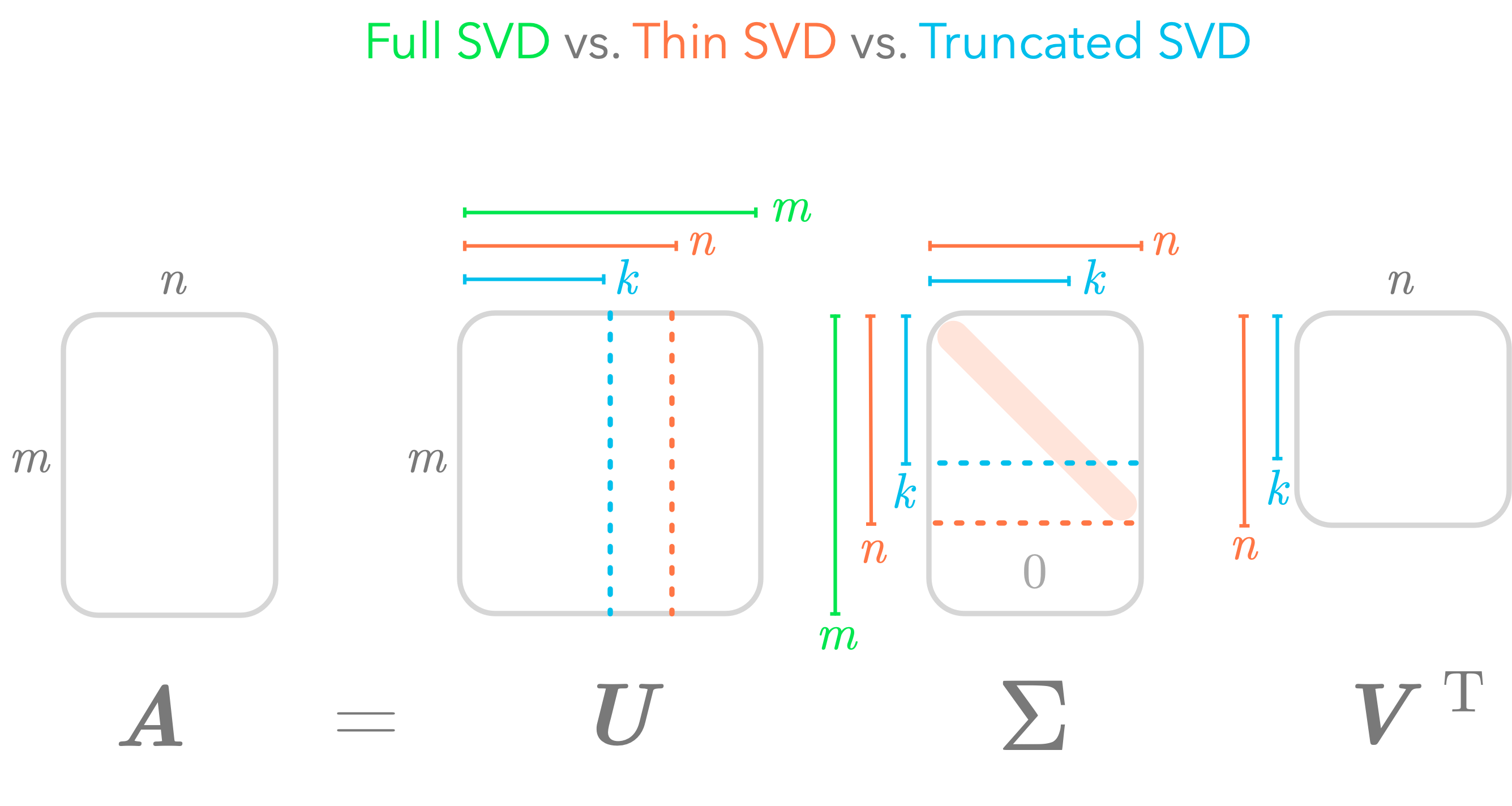Full, Thin and Truncated SVD.

• Ch09. Singular Value Decomposition
• 9.1 Linear Transformation and Change of Basis
• 9.1.1 Linear Transformation
• 9.1.2 Change of Basis
• 9.1.3 Input and Output Bases in Eigendecomposition
• 9.2 Non Square Matrices
• 9.2.1 Different Input and Output Spaces
• 9.2.2 Specifying the Bases
• 9.2.3 Eigendecomposition is Only for Square Matrices
• 9.3 Expression of the SVD
• 9.3.1 From Eigendecomposition to SVD
• 9.3.2 Orthonormal Bases
• 9.3.3 Finding the Singular Vectors and the Singular Values
• 9.4 Geometry of the SVD
• 9.4.1 Summary of the SVD
• 9.4.2 Examples
• 9.4.3 Conclusion
• 9.5 Low-Rank Matrix Approximation
• 9.5.1 Full SVD, Thin SVD and Truncated SVD
• 9.5.2 Decomposition into Rank One Matrices
• 9.6 Hands-On Projects
• 9.6.1 Image Compression
• 9.6.2 PCA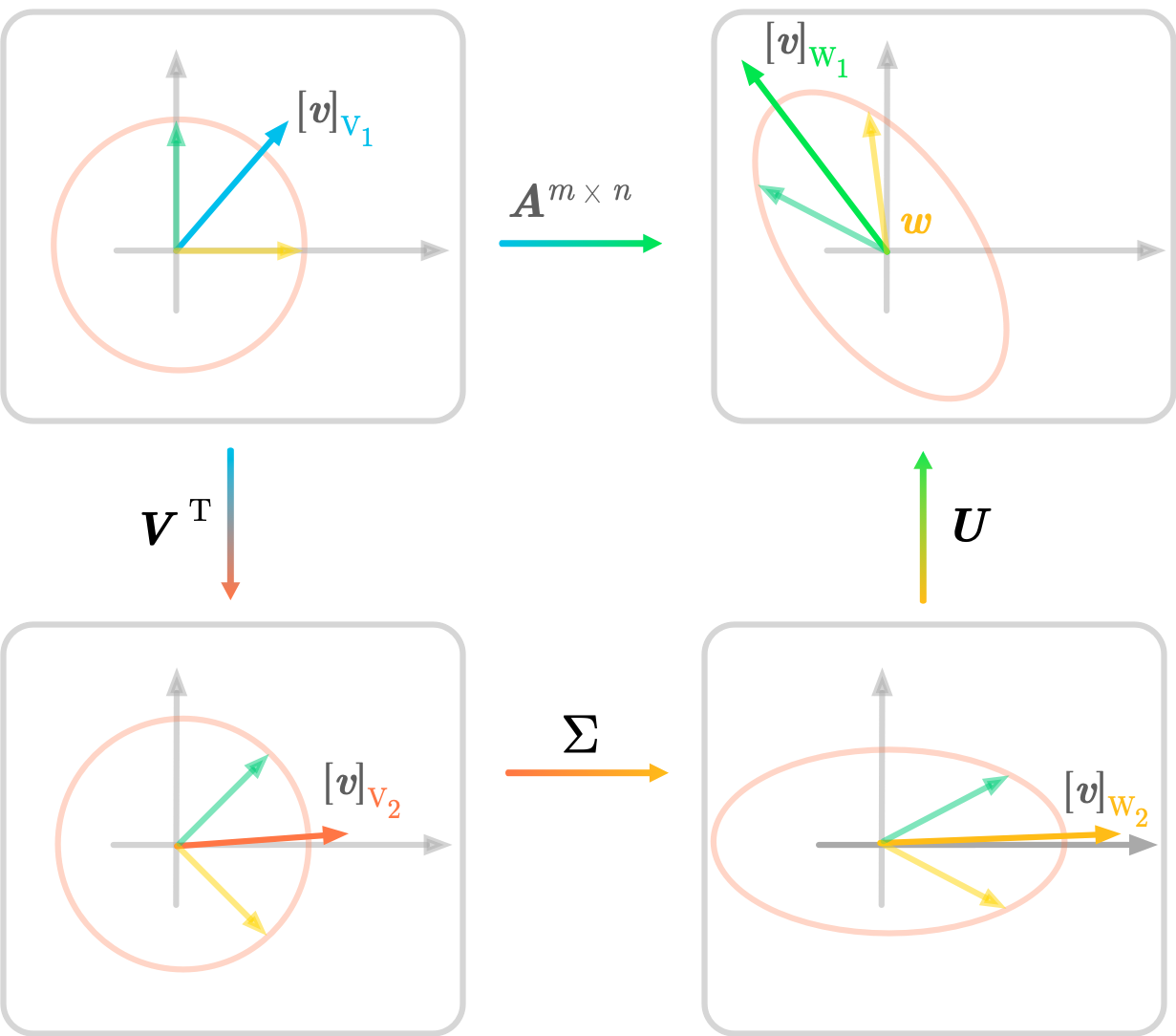SVD Geometry

Part 3 is still in progress and will be about Statistics and Probability. Stay tuned to get the last new about the book!

Feel free to send me your feedbacks/opinions/considerations on this topic, I’ll be very happy to discuss about it!

Essential Math for Data Science
Do you want more math for data science and machine learning? I just released my book "Essential Math for Data Science"🎉.